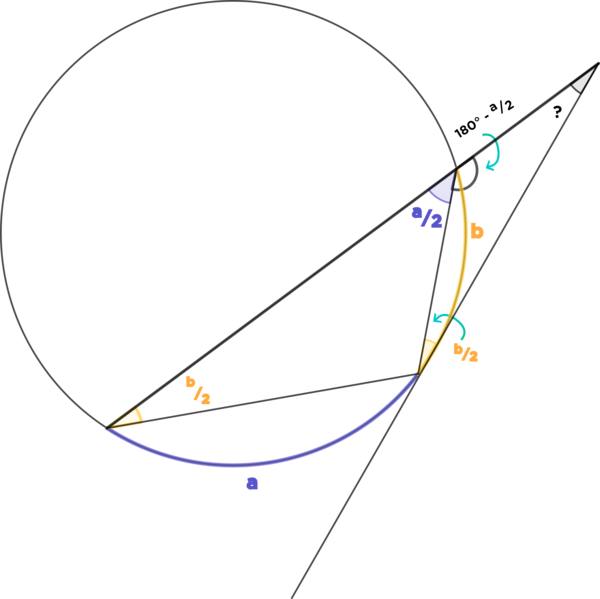# How do we know which angles inscribe which? Do they directly open up to the central angle or something?

• Module 2 Week 2 Day 6 Your Turn Explanation Part 1

How do we know which angles inscribe which? Do they directly open up to the central angle or something?

• @The-Rogue-Blade You can do a shortcut in your head and not worry about the central angle, since the central angle is always the same measure as the arc that it opens up to. So to find the measure of the $$\textcolor{purple}{\text{ angle, }}$$ just find the arc that it opens up to, and take half of it. That's all you do: look for the arc.

Thus $$\textcolor{purple}{\text{ angle } \frac{a}{2}}$$ is half of the measure of $$\textcolor{purple}{\text{ purple arc a,}}$$ and $$\textcolor{orange}{\text{ yellow arc b}}$$ is half of the measure of $$\textcolor{orange}{\text{ yellow arc b.}}$$

When I read your question, this made me think back to the proof of the Inscribed Angle Theorem which, at the time, only had the special case of an angle with one side as the diameter of the circle. So I've added to that proof by giving an additional visual proof of the Inscribed Angle Theorem for general angles, including angles with a tangent line, like the right-hand $$\textcolor{orange}{\text{ yellow }}$$ angle in the diagram.Please check it out!I hope the post with the proof of the Inscribed Angle Theorem will make it more clear why the $$\textcolor{purple}{\text{ purple }}$$ angle has measure $$\textcolor{purple}{\frac{a}{2}}$$ and both $$\textcolor{orange}{\text{ yellow }}$$ angles have measure $$\textcolor{orange}{\frac{b}{2}}$$!